# 2D example: computation of Laplace's electromagnetic force

## Introduction

This section depicts the computation of the mean and pulsating components of Laplace's force in 2D.

## Definition: reminder

The Laplace's electromagnetic force is the force exerted on a conducting conductor placed in a magnetic field.

## Mathematical expression

The force exerted on a conductor can be computed using Laplace's law: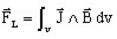where:

•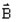is the magnetic flux density in which the conductor is placed
•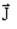is the current density in the conductor

## Expression of the mean and pulsating components of Laplace's force

The magnetic flux density and the current density in a point are expressed in the following way:

• Magnetic flux density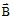is written: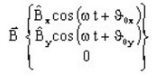That is the parametric equation of an ellipse (see the figure beside)• the current density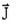is written: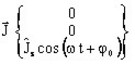Thus , the expression of the Laplace's force in a point is: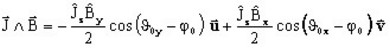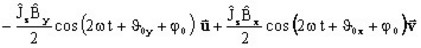## Expression of the mean and pulsating components (continued)

Hence, the Laplace's force is constituted by:

• a mean component :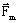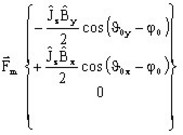• a pulsating component :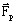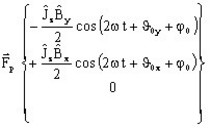## Elliptical representation

The force can be spatially represented as that in the figure below.

• The mean componentis defined by:
• its modulus and its orientation with respect to the Ox axis( α angle)
• The pulsating componentdescribed by an ellipse of pulsation:
• major axis: a
• minor axis: b
• orientation of the ellipse with respect to the Ox (β angle)